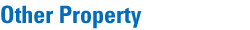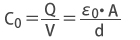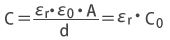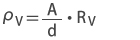Top > Technical Information > Other Property

##### 1. Specific Gravity*(d)

Specific gravity of a glass is defined as the ratio of the glass density to the density of pure water at 4°C and 101.325kPa (1atm.) pressure. Specific gravity is measured using the buoyancy method prescribed in JIS Z 8807:1976.

### 2. Relative Permittivity (εr)

The relative permittivity,εr , is defined by the ratio of the capacitance C/C0. The capacitance C0 of a parallel-plate capacitor in a vacuum is given by the following equation:where V is the potential difference between the plates, Q is the charge on the plates, ε0 is the
permittivity of vacuum ( = 8.854×10-12 F/m), A is the area of the plates and d is the distance between the plates.

When an insulator is introduced between the plates of a capacitor, the capacitance increases and its value, C, is given by the following equation:εr in the above equation is called the relative permittivity which can be obtained from the ratio C/C0.
In this catalog, it is measured at 20°C and at 1MHz by the method specified in JIS C 2141-1978.

### 3. Volume Resistivity (ρV)

The volume resistance between the two electrodes forming the two opposite sides of a cube with 1cm edges is called the volume resistivity, ρV, or simply the resistivity. In this catalog the volume
resistance, RV (Ω), is measured with D.C.500V by the method specified in JIS C 2141-1978 and the volume resistivity, ρV (Ω, cm), is calculated by the following equation:where A is the effective area (cm2) of the main electrode of the disklike specimen and d is the thickness (cm) of the specimen. In this catalog, the volume resistivity is measured at 20°C and 200°C and the measured values are expressed in terms of ρV20°C and ρV200°C.

It is measured by the method of applying to JIS C2139:2008.

Optical Glass Permittivity, Volume Resistivity Measurement Example

Glass Permittivity Volume Resistivity 20°C Volume Resistivity 200°C
ρV20 (Ω, cm) ρV200 (Ω, cm)
FC5 5.6 1.0×1015 4.1×1015
FCD1 9.3 2.0×1015 1.5×1012
FCD10 9.1 8.0×1015 6.0×1010
PCD4 9.0 0.9×1015 0.7×1014
BSC7 5.8 6.0×1014 2.5×1011
BAC4 7.0 1.0×1015 2.0×1011
BACD5 6.8 1.8×1016 0.7×1015
BACD15 8.1 2.5×1015 1.7×1015
LAC8 8.7 1.0×1015 1.0×1015
LAC14 9.1 3.3×1016 4.2×1015
TAC8 9.8 2.8×1016 1.7×1015
BAF10 9.0 1.0×1015 1.2×1014
E-FL6 7.5 6.7×1013 1.2×108
E-FDS1 22.8 1.0×1016 5.1×1011
FDS18 25.6 5.3×1014 1.4×1010
LAF2 11.0 1.0×1015 1.0×1015
NBF1 9.9 3.4×1016 0.8×1015
TAF1 10.9 5.0×1016 1.3×1015
TAF3 11.8 2.7×1015 1.3×1015
NBFD10 12.0 1.0×1015 1.0×1015
NBFD13 12.0 1.0×1015 1.0×1015
TAFD5F 13.8 3.4×1016 4.2×1014
TAFD25 16.9 2.3×1016 4.3×1014
TAFD30 14.4 2.5×1016 4.7×1011
TAFD40 20.5 1.9×1016 7.7×1014
LBC3N 8.8 9.7×1015 8.4×1010